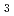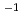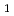# Evaluation of required activity of SOdecomposition catalyst for iodine-sulfur process
Required performance of SOdecomposition catalyst for Iodine-Sulfur process was investigated. Heat transfer area needed for shell and tube type SOdecomposer exchanging heat from VHTR was calculated by applying Yagi-Kunii and Zukauskas's equation for filled layer-SiC tube and SiC tube-He gas flow heat transfer respectively and the minimum space velocity for catalyst was 1000 h. To transform minimum space velocity to more universal kinetic rate constant, we introduced forward/reverse SOdecomposition equation. To achieve equilibrium SOdecomposition ratio above 0.5 MPa, rate constant kshould be more than 1.5 sfor SOdecomposition catalyst.